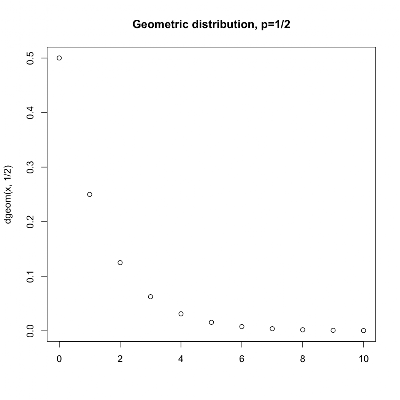## Sunday, March 13, 2011

### Using R for Introductory Statistics, The Geometric distribution

We've already seen two discrete probability distributions, the binomial and the hypergeometric. The binomial distribution describes the number of successes in a series of independent trials with replacement. The hypergeometric distribution describes the number of successes in a series of independent trials without replacement. Chapter 6 of Using R introduces the geometric distribution - the time to first success in a series of independent trials.Specifically, the probability the first success occurs after k failures is:Note that this formulation is consistent with R's [r|d|p|q]geom functions, while the book defines the distribution slightly differently as the probability that the first success occurs on the kth trial, changing the formula to:We'll use the first formula, so k ∈ 0,1,2,..., where 0 means no failures - success on the first try. The intuition is that the probability of failure is (1-p), so the probability of k failure is (1-p) to the kth power.

Let's generate 100 random samplings where the probability of success on any given trial is 1/2, like we were repeatedly flipping a coin and recording how many heads we got before we got a tail.

```> sample <- rgeom(100, 1/2)
> summary(sample)
Min. 1st Qu.  Median    Mean 3rd Qu.    Max.
0.0     0.0     0.0     0.9     1.0     5.0
> sd(sample)
 1.184922
> hist(sample, breaks=seq(-0.5,6.5, 1), col='light grey', border='grey', xlab="")
```As expected, we get success on the first try about half the time, and the frequency drops in half for every increment of k after that.The median is 0, because about 1/2 the samples are 0. The mean is, of course, higher because of the one-sidedness of the distribution. The mean of our sample is 0.9, which is not too far from the expected value of 1. Likewise, the standard deviation is not far from the theoretical value of √2 or 1.414214.

This is part of an ultra-slow-motion reading of John Verzani's Using R for Introductory Statistics. Notes on previous chapters can be found here:

Chapters 1 and 2

Chapter 3

Chapter 4

Chapter 5

#### 1 comment:

1."The mean is, of course, higher because of the one-sidedness of the distribution"

I think you mean to say the mean is higher because it's right skewed.